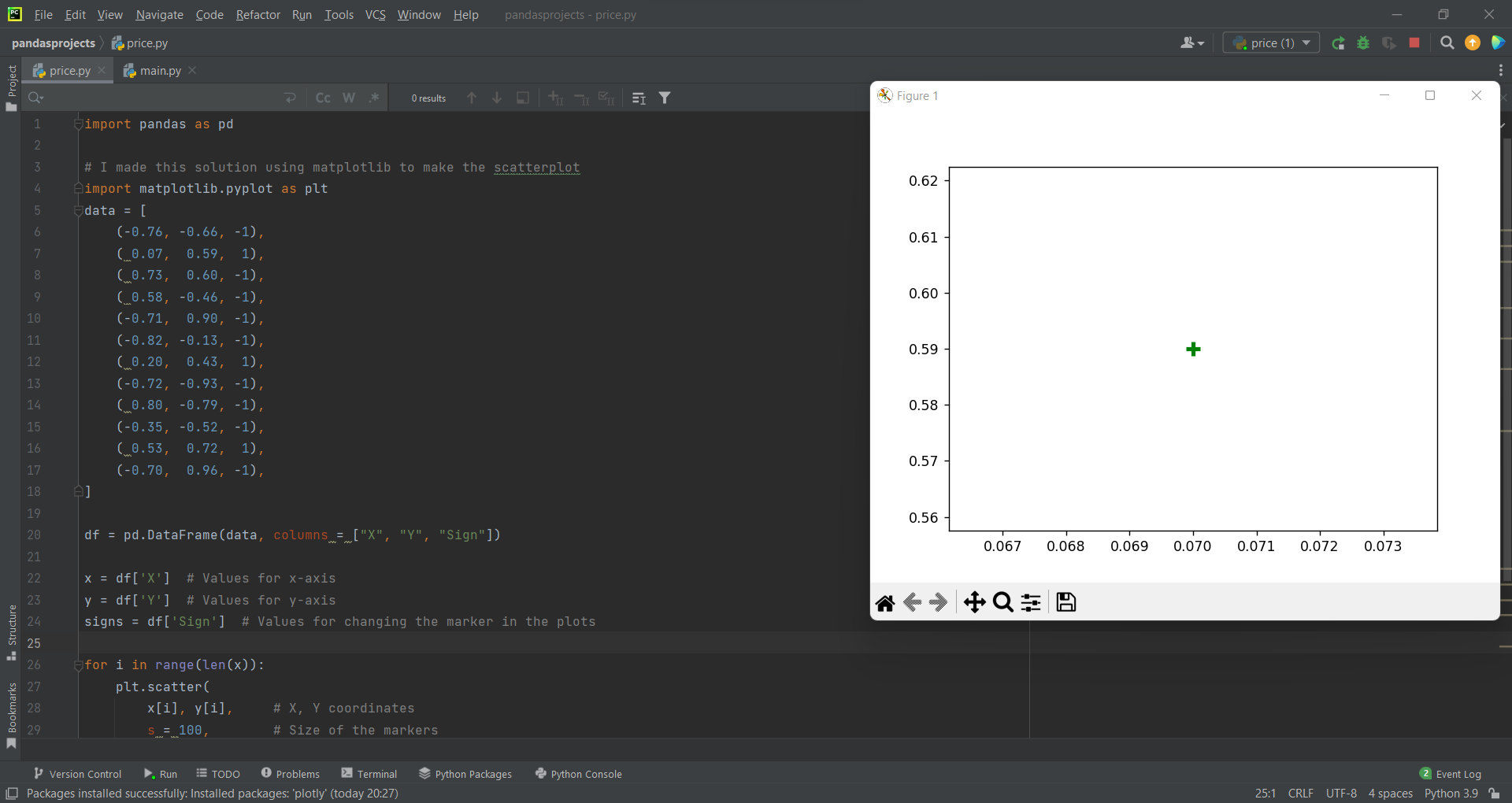# Plot and Scatter using Pandas in Pythonby Abdul Rawoof A RUpdated: Feb 20, 2023Solution Kit

Plotting is nothing, but it draws points or markers in a diagram. The plot() function will draw a line from point to point, and this function takes parameters for specifying points in the diagram. Scatter is a diagram where a dot represents each value in the data set.

To create a scatterplot using data from a pandas DataFrame, we have two different ways,

• The first way to create a scatterplot is to use the built-in pandas plot.scatter function and import pandas library as import pandas as pd df.
• And the second way is to use the matplotlib.pyplot.scatter.

In scatter plots, identification and correlational relationships are common. The main use of a scatter plot is to observe and show the relationship between numeric variables and the dots in a scatter plot report not only the values of individual data points in pandas but also patterns when the data or information are taken as a whole. The best type of data for scatter plots is when we have two variables that pair well together, and if we have two variables which will pair well together and plotting them in a scatter diagram is a great way to view their relationship and then see if it's a positive or negative correlation. The disadvantage of the scatter plot is it cannot provide the precise extent of association, and a scatter plot does not indicate the quantitative measure of the relationship between the two variables.

Here is an example of how you can plot and scatter using pandas in Python:Fig : Preview of the output that you will get on running this code from your IDE.

### Code

In this solution we're using Pandas library.

### Instructions

Follow the steps carefully to get the output easily.

2. Copy the snippet using the 'copy' and paste it in your IDE.
3. Add required dependencies and import them in Python file.
4. Run the file to generate the output.

I hope you found this useful. I have added the link to dependent libraries, version information in the following sections.

I found this code snippet by searching for 'plot scatter using pandas' in kandi. You can try any such use case!

### Environment Tested

I tested this solution in the following versions. Be mindful of changes when working with other versions.

1. The solution is created in PyCharm 2021.3.
2. The solution is tested on Python 3.9.7.
3. Pandas version-v1.5.2.

Using this solution, we are able to plot and scatter using pandas in python with simple steps. This process also facilities an easy way to use, hassle-free method to create a hands-on working version of code which would help us to plot and scatter using pandas in python.

### Dependent Library

pandasby pandas-dev

Python38689Version:v2.0.2Flexible and powerful data analysis / manipulation library for Python, providing labeled data structures similar to R data.frame objects, statistical functions, and much more

Support
Quality
Security
Reuse

pandasby pandas-dev

Python38689Version:v2.0.2License: Permissive (BSD-3-Clause)

Flexible and powerful data analysis / manipulation library for Python, providing labeled data structures similar to R data.frame objects, statistical functions, and much more
Support
Quality
Security